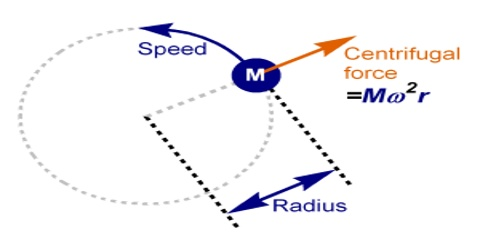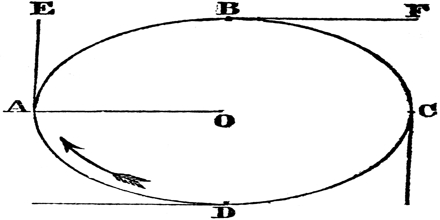Physics

# Centrifugal ForceCentrifugal force

We know that for every body moving in a circular path a force act towards the centre of the circle i.e., a centripetal force acts. The earth rotates around the sun. Here gravitational attraction force of the sun on the earth is the centripetal force. Naturally question may arise- by which force the centripetal force is balanced? For what hindrance the earth does not go straight to the sun? Apparently, it appears that another equal and opposite force acts on the earth. This apparent force is called centrifugal force.

Obviously, centrifugal force is equal and opposite to the centripetal force. But it should be remembered that there is no real existence of centrifugal force. So actually the centripetal force is not balanced by any real force Centrifugal force is an imaginary force.For everybody rotating in a circular orbit has a tendency to move away along the tangent of the circle, for example, in case of rotation of a stone by a thread if the thread is turn off then the thread speeds away along the tangent of the circle. Suppose, a body moving in a circular path is at position ‘A’ at any instant [Figure]. If no centripetal force acts along the direction of the centre of the circle O, then the body would have reached to point ‘B’ along the tangent. But since the centripetal force acts, so the body approaches slightly towards the centre and reaches to point C. a point in the circle, instead of point B. That means, due to the influence of centripetal force the body is forced to move at every moment along the circular path. So, the centripetal force is always active in rotational motion.

Hence, the centripetal force as not balance by any real force. For this reason, the presence of centrifugal force is apparently considered as real which acts opposite to the centripetal force. So, it is called pseudo force.

The imaginary force which is equal and opposite to the, centripetal force acting on a body moving in circular orbit with uniform speed is ailed centrifugal force. It acts opposite to radius vector outwardly.